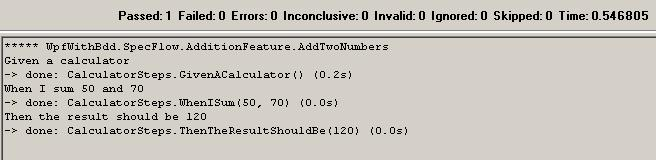Article Image

I have heard a lot of nice things about BDD (Behavior Driven Development), so today I have decided to try out SpecFlow. I have downloaded and installed. Then I have created a new/empty WPF application and added a very simple calculator interface (with one sum method) and implemented.

For BDD testing I haved added a new project to my solution with a simple SpecFlow feature as follows:

``````Feature: Addition
In order to avoid silly mistakes
As a math idiot
I want to be told the sum of two numbers

Given a calculator
When I sum 50 and 70
Then the result should be 120
``````

Then I have added a new class to my test project and implemented the steps for testing as follows:

``````[Binding]
class CalculatorSteps
{
private readonly StandardKernel _kernel = new StandardKernel();

private ICalculator _calc;
private int _lastResult;

public CalculatorSteps()
{
_kernel.Bind<ICalculator>().To<Calculator>();
}

[Given(@"a calculator")]
public void GivenACalculator()
{
_calc = _kernel.Get<ICalculator>();
}

[When(@"I sum (\d+) and (\d+)")]
public void WhenISum(int num1, int num2)
{
_lastResult = _calc.Sum(num1, num2);
}

[Then(@"the result should be (\d+)")]
public void ThenTheResultShouldBe(int result)
{
Assert.AreEqual(result, _lastResult);
}
}
``````

Note: As you see I am using Ninject (StandardKernel), but there is no real advantage of using an IoC container for this task, it's just for fun and because I like to learn/study Ninject in the near future.

Finally build the solution, open the test assembly (WpfWithBdd.SpecFlow.dll) with NUnit and hit Run... producing the following output:The output is nice and self-explanatory!

The very trivial code for this post is available here.

Hope you enjoy. See you next time.

Image